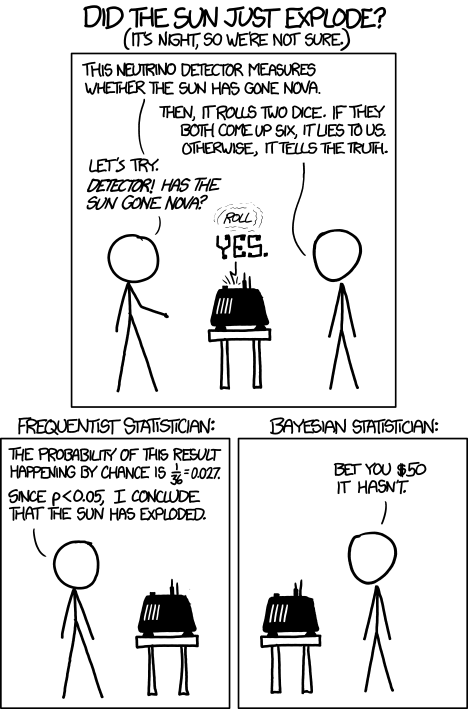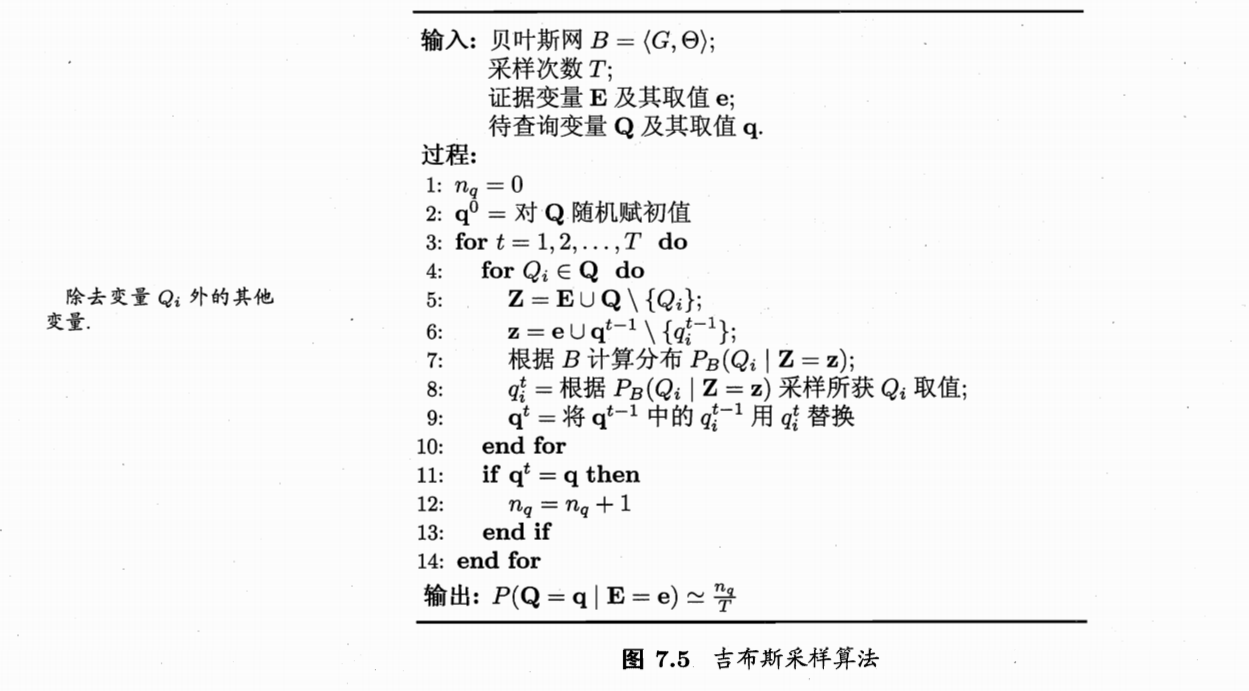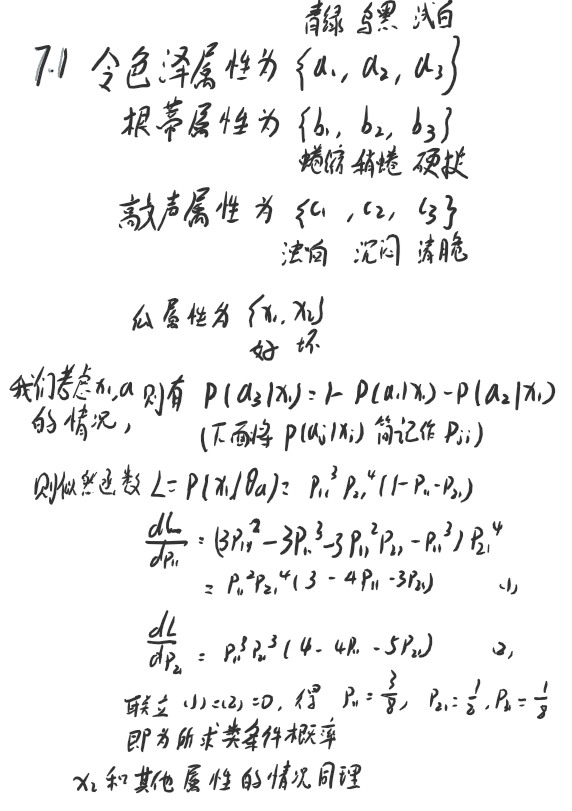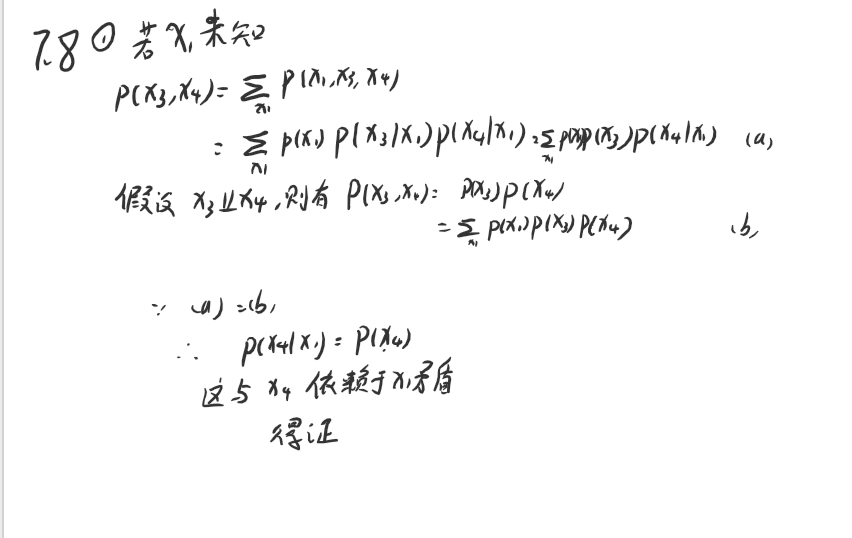# 极大似然估计

1. 训练样本不足，导致数据稀疏
2. 特征较多，产生组合爆炸，计算复杂性出问题

# 半朴素贝叶斯分类器

## TAN

TAN(Tree Augmented naive Bayes)算法则是在最大带权生成树算法基础上，将属性依赖关系通过以下步骤约简为树形结构：

1. 计算任意两个属性之间的条件互信息（conditional mutual information）:
2. 以属性为节点构建完全图，权重为$I(x_i,x_j|y)$
3. 构建此完全图的最大带权生成树，挑选根变量，置边为有向
4. 加入类别节点，增加从类别节点到各个属性的有向边
TAN实际保留了强相关属性之间的依赖性。

## AODE

AODE(Averaged One-Dependent Estimator)算法是一种基于集成学习的更强的独依赖分类器，AODE尝试将每个属性都作为超父来构建SPODE,然后将具有足够训练数据支持的SPODE集成起来作为最终结果。即：

## 贝叶斯网

### 结构

• 条件独立
若对给定的$a$　的取值 ，$p(b,c|a)=p(b|a)p(c|a)$,我们称之为条件独立
• 边际独立
若 $a$ 的取值完全未知，而$b,c$ 此时相互独立　$p(b,c)=p(b)p(c)$　，我们称之为边际独立

这里补充一下概率论的一些常见误区：
独立性不能导出条件独立性，一个很直观的例子，随机变量Ａ表示硬币１向上的事件，Ｂ表示硬币２向上，显然Ａ和Ｂ是独立的，但是给出随机变量Ｃ表示硬币１，２是否同向，那么对于给定的Ｃ，Ａ和Ｂ显然不是条件独立的。

### 学习

“评分搜索“是一种寻找网络结构的常见方法。

### 推断吉布斯采样

## EM算法

Em算法（Expectation-Maximization），即期望最大化算法常用于估计参数隐变量。基本思想是，若参数 $\Theta$ 已知，则推断出最优隐变量(E步)，若$Z$ 已知，则对参数做极大似然估计(M步)。

• E(Expectation):
以当前参数$\Theta^t$ 推断隐变量分布，$P(ZZ|X,\Theta_t)$，并计算对数似然　$LL(\Theta|X,Z)$　关于$Z$ 的期望，
• M(Maximization):
寻找参数最大化期望似然，即

# 习题与答案

7.1 试使用极大似然法估算西瓜数据集3.0中前3个属性的类条件概率。7.2 试证明：条件独立性假设不成立时，朴素贝叶斯分类器仍有可能产生最优贝叶斯分类器。

7.3 试编程实现拉普拉斯修正的朴素贝叶斯分类器，并以西瓜数据集3.0为训练集，对p.151“测1”样本进行判别。

def calContProbDistrete(data, feature):
"""
Data - dataframe contain two colunms, the given feature of samples and it label
"""
prob = pd.DataFrame(0, columns=data['label'].unique(), index=data[feature].unique())
ni = len(data[feature].unique())
prob = np.zeros((len(data['label'].unique()), len(data[feature].unique())) )

for i in data['label'].unique():
nc = len(data[data['label'] == i]) + ni
for j in data[feature].unique():
prob[i,j] = (len(data[data['label']==i][data[feature]==j]) + 1) / nc

return prob


7.4 实践中使用式（7.5）决定分类类别时，若数据的维数非常高，则概率连乘的结果通常会非常接近于0从而导致下溢。试述防止下溢的可能方案。

7.5 试证明：二分类任务中两类数据满足高斯分布且方差相同时，线性判别分析产生贝叶斯最有分类器。

$\frac{1}{J}$的最小值。这里$\sum_i$代表的协方差矩阵由于特征之间的独立性，是个对角矩阵，那么这里的 $w^T\sum_1w$ 的二次型就可以看作是二范数，那么就有：

7.6

7.7 给定 $d$ 个二值属性的二分类任务，假设对于任何先验概率项的估算至少需要30个样例，则在朴素贝叶斯分类器式（7.15）中估算先验概率项需要60个样例。试估计在AOED式中估算先验概率项所需的样例数。（分别考虑最好和最坏情况）

7.87.8